« One-storey plane frames The degree of fixity effect of columns » Bending and shearing effect on deformations and stresses It is known from the mechanics that the deformation of a member depends on the works done by bending moments and shear forces. The stiffness E ·I of earthquake resistant structural elements (cracked cross-sections) is half of the respective stiffness of uncracked cross-sections [EC8, §4.3.1(6),(7)]. The shear modulus G, on which the shear deformation depends, is related to the modulus of elasticity E and Poisson factor ν by the following expression,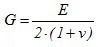In EC2 it is assumed that for a cracked cross section ν=0 and for an uncracked cross-section ν=0.2 → Gvaries from 0.5E to 0.42E. In calculations for earthquake resistance it is recommended to assumeν =0 and therefore G=0.5E. Fixed-ended column A column fixed at both ends bearing at the top a mass m, will be now considered. Gravity acceleration causes a vertical load W=g·m. Due to seismic acceleration a, a horizontal forceH=a· m[=(a/g)·W is developed at the column top resulting its relative displacement by δ.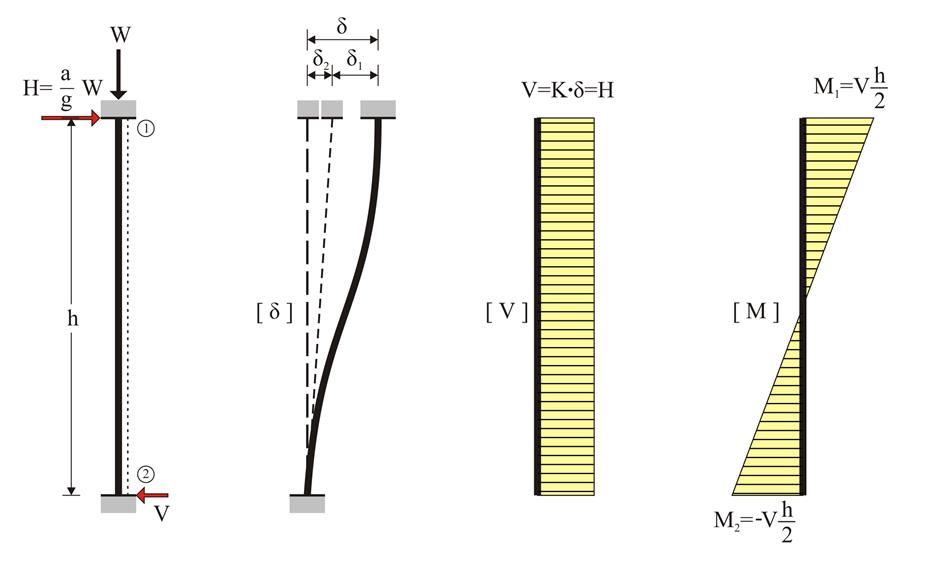Figure 5.1.1: Fixed-ended column: K = (12 E I / h 3) k va Figure 5.1.1: Fixed-ended column: K = (12 E I / h 3) k va The total displacement δ is due to: (a) the displacement δ1 due to bending caused by force H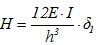(b) the displacement δ2 due to shearing caused by force H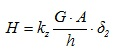where kz is the correction factor of the shear effect, G is the concrete shear modulus and A the concrete cross-section. δ = δ1+δ2 →In conclusion, the horizontal seismic force Η is related to the corresponding displacement δ by the following expression: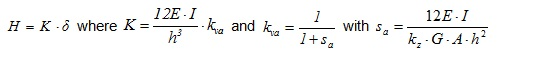The term K denotes the stiffness of the fixed-ended column. kva is the correction factor of the bending stiffness of the fixed-ended column due to shearing. Always kva <1.0. It can be ignored for ordinary columns (i.e. it may be assumed that kva=1.0). On thecontrary, in the case of to walls it must be taken into account since its value is significantly lower than 1.0. Indicatively for walls with length 1.0 m and 2.0 m, kva is equal to 0.80 and 0.50 respectively. Example 5.1.1-1 : Consider a fixed-ended column with a cross section of 400m m /400 mm , height h =3.0 m , elasticity modulus E =32.8 GP , bearing a concentrated mass of m =80 t at the top. The design seismic acceleration factor at the top is a / g =0.10. Calculate the stress resultants and the relative displacement at the top. W =80 · 1000 kg · 10 m / sec 2 =800 kN , H =0.10·800=80 kN , V = H =80 kN M 1 =- Μ 2 =- H · h /2=-80·3.0/2=120 kNm , I =0.40·0.403/12=21.33·10-4 m 4 Without shear effect, K=12 · EI / h 3 =12 · 32.8 · 109 · Ν /m2 · 21.33 · 10-4m4/(3.03 · m3)=31.10·106 N / m With shear effect, for G=0.5E, kz=5/6 (rectangular cross-section) and A=b · d: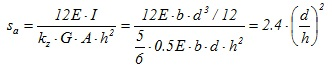This expression is independent of the cross-sectional width and applies for all fixed-ended rectangular columns. For this column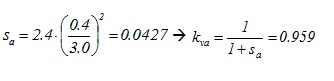therefore K = (E· I / h3)· Kva =31.10·106·0.959 N/m =29.82·106 N/m. In conclusion: Without shear effect, δ =H/K=80 · 103N/(31.10 · 106N/ m ) =2.57 mm . With shear effect, δ =H/K=80 · 103N/(29.82 · 106N/ m ) =2.68 mm , i.e. the displacement is 4% higher than the respective value without shear effect. Example 5.1.1-2 Consider the same data and questions of the previous example for a wall with cross-section 2000/300. W =80 · 1000 kg · 10 m / sec 2 =800 kN , H =0.10·800=80 kN , V = H =80 kN M 1 =- Μ 2 =- H · h /2=-80·3.0/2=120 kNm , I =0.30·2.003/12=0.20 m 4 Without shear effect, K=12 · EI / h 3 =12 · 32.8 · 109 · Ν /m2 · 0.20m4/(3.03 · m3)=2915.56·106 N / m For this column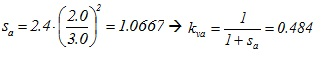therefore K = (E· I / h3)· Kva =2915.56·106·0.484 N / m =1410.75·106 N / m In conclusion: Without shear effect, δ =H/K=80 · 103N/(2915.56 · 106N/ m ) =0.027 mm . With shear effect, δ =H/K=80 · 103N/(1410.75 · 106N/ m ) =0.057 mm , i.e. the displacement is 110% higher than the respective value without shear effect. « One-storey plane frames The degree of fixity effect of columns »